### Home > CALC > Chapter 11 > Lesson 11.1.3 > Problem11-35

11-35.
1. If f(x) = g(h(x)), then: Homework Help ✎

1. Explain what each of these expressions represent: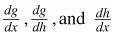.

2. Explain why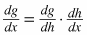.

3. Solvefor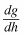.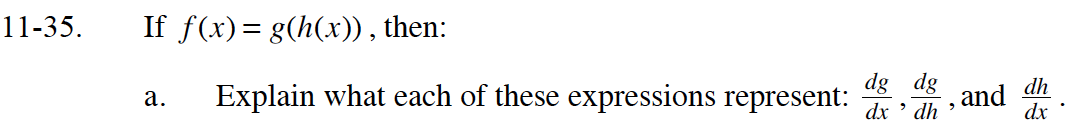dg/dx is the rate of change of g with respect to x.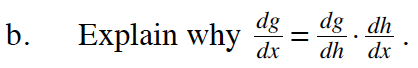Since g is a function of h and h is a function of x, __________________.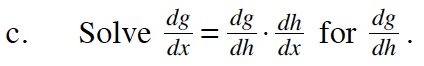Multiply both sides of the equation by dx/dh.